# Civil Engineering - UPSC Civil Service Exam Questions

21.

Two springs of stiffnesses KA and KB are placed on inside the other so as to compressed by the same amount under an applied axial load. The combined stiffness of the two springs will be

 A.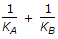B. KA KB/(KA + KB) C. (KA + KB)/2 D. KA + KB

Explanation:

No answer description available for this question. Let us discuss.

22.

If the Young's modulus 'E' is equal to bulk modulus 'K', then what is the value of the Posson's ratio ?

 A.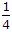B.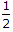C.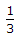D.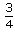Explanation:

No answer description available for this question. Let us discuss.

23.

At the time of initial tensioning the maximum tensile stress in tendon immediately behind the anchorage shall Not exceed

 A. 50% of the ultimate tensile strength of the wire or bar strength B. 80% of the ultimate tensile strength of the wire or bar strength C. 40% of the ultimate tensile strength of the wire or bar strength D. 60% of the ultimate tensile strength of the wire strength

Explanation:

No answer description available for this question. Let us discuss.

24.

The following statements relate to a laminar flow :
1. Laminar flow is rotational.
2. In laminar flow the loss of head is proportional to the square of the velocity.
3. In laminar flow the loss of head is proportional to the first power of viscosity.
4. In laminar flow the velocity is constant over the cross-section.
5. Other quantities remaining the same, increase in diameter will increase the Reynolds number in laminar flow.
Of these statements :

 A. 1, 2 and 4 are correct B. 1, 3 and 4 are correct C. 1, 3 and 5 are correct D. 2, 3 and 5 are correct

Explanation:

No answer description available for this question. Let us discuss.

25.

Plate load test is useful to estimate

 A. Bearing capacity of foundation B. Settlement of foundation C. Both bearing capacity and settlement of foundation D. Depth of foundation